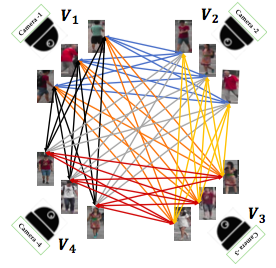## neal young / Roy18Exploiting

•Minimization of labeling effort for person re-identification in camera networks is an important problem as most of the existing popular methods are supervised and they require large amount of manual annotations, acquiring which is a tedious job. In this work, we focus on this labeling effort minimization problem and approach it as a subset selection task where the objective is to select an optimal subset of image-pairs for labeling without compromising performance. Towards this goal, our proposed scheme first represents any camera network (with k number of cameras) as an edge weighted complete k-partite graph where each vertex denotes a person and similarity scores between persons are used as edge-weights. Then in the second stage, our algorithm selects an optimal subset of pairs by solving a triangle free subgraph maximization problem on the k-partite graph. This sub-graph weight maximization problem is NP-hard (at least for k larger than 3) which means for large datasets the optimization problem becomes intractable. In order to make our framework scalable, we propose two polynomial time approximately-optimal algorithms. The first algorithm is a 1/2-approximation algorithm which runs in linear time in the number of edges. The second algorithm is a greedy algorithm with sub-quadratic (in number of edges) time-complexity. Experiments on three state-of-the-art datasets depict that the proposed approach requires on an average only 8-15% manually labeled pairs in order to achieve the performance when all the pairs are manually annotated.